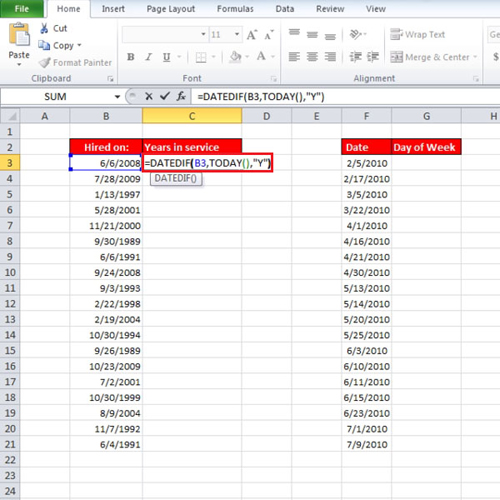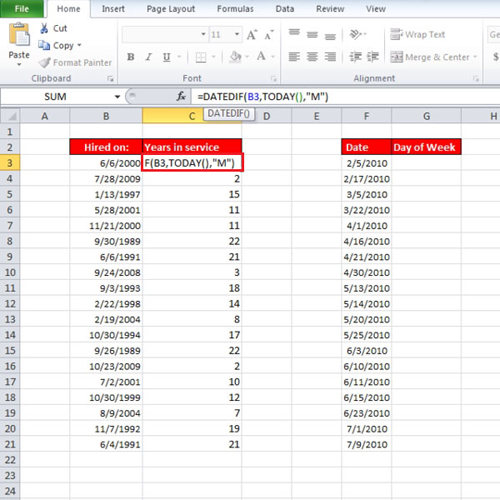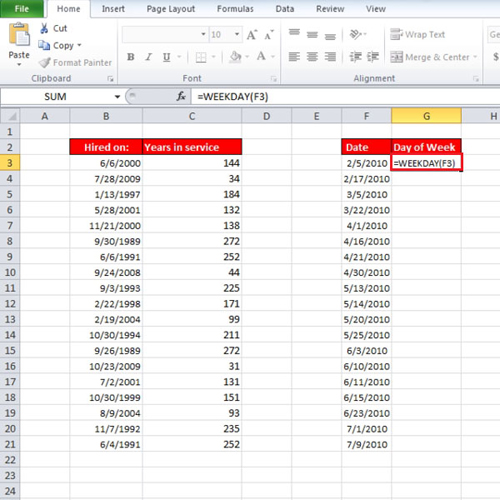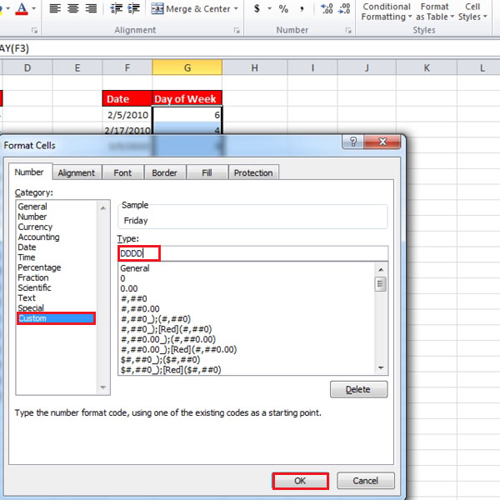## How to calculate date differences by year, month, day, and determine the day of the week in Excel

In this tutorial, we will teach you how to calculate date difference by year, month or day in Excel. In this step by step guide, we will teach you two date functions. The first one calculates the time elapsed and the second one allows you to find a specific day of the week. Such functions are very useful when calculating date differences.

Step # 1 – Calculate time elapsed
In this tutorial, we have a list of dates on which people were hired. So in order to calculate their years in service, write:
= datedif (b3, today(), “y” )
In the tutorial, since the date on which the employee was hired is in column “b” and the first date starts with row “3” that is why it was used in the function. You can use the autofill option to fill up the rest of the columns. If you change the date then the years in service will be recalculated.Step # 2 – Modify the datedif function
If you replace “y” with “m” in the date function, the number of months will be calculated and if you replace it with “d” then number of days will be calculated.Step # 3 – Apply the weekday function
Now the other date function is for determining a specific day of the week. Write the function
=weekday(f3)Step # 4 – Format Cells for Day Name
When you press the “enter” key, you will see the number instead of the name of the day. First fill up the entire column and then right click on it and select “format cell”. Over there, under the “number” tab select “custom” and write “dddd”. Press the “ok” button to exit.